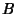## Pick's Theorem

Letbe the Area of a simply closed Polygon whose Vertices are lattice points. Letdenote the number of Lattice Points on the Edges andthe number of points in the interior of the Polygon. ThenThe Formula has been generalized to 3-D and higher dimensions using Ehrhart Polynomials.

See also Blichfeldt's Theorem, Ehrhart Polynomial, Lattice Point, Minkowski Convex Body Theorem

References

Diaz, R. and Robins, S. Pick's Formula via the Weierstraß-Function.'' Amer. Math. Monthly 102, 431-437, 1995.

Ewald, G. Combinatorial Convexity and Algebraic Geometry. New York: Springer-Verlag, 1996.

Hammer, J. Unsolved Problems Concerning Lattice Points. London: Pitman, 1977.

Morelli, R. Pick's Theorem and the Todd Class of a Toric Variety.'' Adv. Math. 100, 183-231, 1993.

Pick, G. Geometrisches zur Zahlentheorie.'' Sitzenber. Lotos (Prague) 19, 311-319, 1899.

Steinhaus, H. Mathematical Snapshots, 3rd American ed. New York: Oxford University Press, pp. 97-98, 1983.